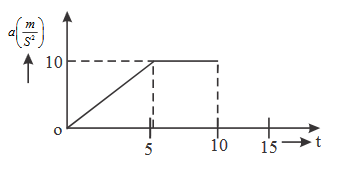21.  Rohit completes a semi circular path of radius R in 10 seconds. Calculate average speed and average velocity in m/s.

A. $$\frac{2\pi R}{10},\frac{2R}{10}$$

B. $$\frac{\pi R}{10},\frac{R}{10}$$

C. $$\frac{\pi R}{10},\frac{2R}{10}$$

D. $$\frac{2\pi R}{10},\frac{R}{10}$$

22.  A particle moves 4m in the south direction. Then it moves 3m in the west direction. The time taken by the particle is 2 second. What is the ratio between average speed and average velocity ?

A. $$\frac{5}{7}$$

B. $$\frac{7}{5}$$

C. $$\frac{14}{5}$$

D. $$\frac{5}{14}$$

23.  A particle is projected vertically upwards with velocity 30 m/s. Find the ratio of average speed and instantaneous velocity after 6 s. $$\left [ g=10\ ms^{-2} \right ]$$

A. $$\frac{1}{2}$$

B. 2

C. 3

D. 4

24.  The motion of a particle along a straight line is described by the function $$x=\left ( 3t-2 \right )^{2}$$. Calculate the acceleration after 10s.

A. $$9\ ms^{-2}$$

B. $$18\ ms^{-2}$$

C. $$36\ ms^{-2}$$

D. $$6\ ms^{-2}$$

Explanation :

$$v=\frac{\mathrm{d} x}{\mathrm{d} t}$$ and $$a=\frac{\mathrm{d} v}{\mathrm{d} t}$$

25.

Given figure shows a graph at acceleration $$\rightarrow$$ time for a rectilinear motion. Find averageacceleration in first 10 seconds.A. $$10\ ms^{-2}$$

B. $$15\ ms^{-2}$$

C. $$7.5\ ms^{-2}$$

D. $$30\ ms^{-2}$$

26.  A body starts its motion with zero velocity and its acceleration is $$3\ ms^{-2}$$. Find the distance traveled by it in fifth second.

A. 15.5 m

B. 17.5 m

C. 13.5 m

D. 14.5 m

27.  A body is moving in x direction with constant acceleration $$\alpha$$. Find the difference of the displacement covered by it in nth second and $$(n-1)^{th}$$ second.

A. $$\alpha$$

B. $$\frac{\alpha }{2}$$

C. $$3\alpha$$

D. $$\frac{3\alpha }{2}$$

28.  What does the speedometer measure kept in motorbike ?

A. Average velocity

B. Average speed

C. Instantaneous speed

D. Instantaneous velocity

29.  The displacement of a particle in x direction is given by $$x=9-5t+4t^{2}$$. Find the velocity at time $$t = 0$$

A. -8 m/s

B. -5 m/s

C. 3 m/s

D. 10 m/s

30.  A freely falling particle covers a building of 45m height in one second. Find the height of the point from where the particle was released. $$\left [ g=10\ ms^{-2} \right ]$$